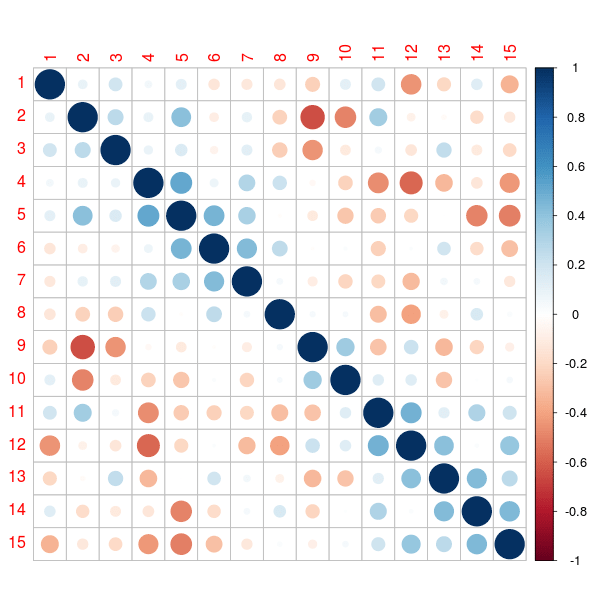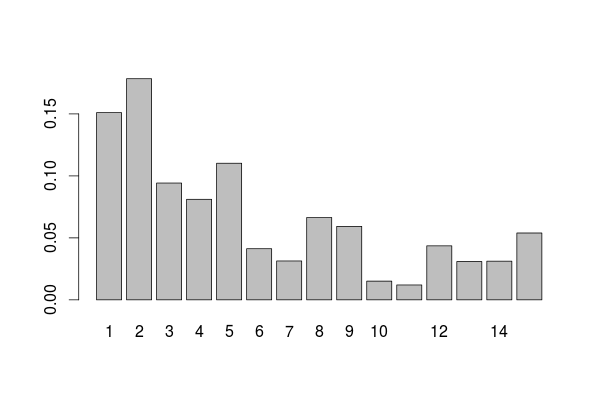Want to share your content on R-bloggers? click here if you have a blog, or here if you don't.

Classification trees are nice. They provide an interesting alternative to a logistic regression.  I started to include them in my courses maybe 7 or 8 years ago. The question is nice (how to get an optimal partition), the algorithmic procedure is nice (the trick of splitting according to one variable, and only one, at each node, and then to move forward, never backward), and the visual output is just perfect (with that tree structure). But the prediction can be rather poor. The performance of that algorithme can hardly compete with a (well specified) logistic regression.

Then I discovered forests (see Leo Breiman’s page for a detailed presentation). Being a huge fan of boostrap procedures I loved the idea. In regression models, I usually mention boostrap to avoid asymptotic approximations: we boostrap the rows (the observations). In the case of random forest, I have to admit that the idea of selecting randomly a set of possible variables at each node is very clever. The performance is much better, but interpretation is usually more difficult. And something that I love when there are a lot of covariance, the variable importance plot. Which is something that we can hardly get with econometric models (please let me know if I’m wrong).

In order to illustrate, let us generate a large dataset. Not necessarily huge, but large, so that we really have to select variables.  Since it is more interesting if we have possibly correlated variables, we need a covariance matrix. There is a nice package in R to randomly generate covariance matrices.

> set.seed(1)
> n=500
> library(clusterGeneration)
> library(mnormt)
> S=genPositiveDefMat("eigen",dim=15)
> S=genPositiveDefMat("unifcorrmat",dim=15)
> X=rmnorm(n,varcov=S$Sigma) > library(corrplot) > corrplot(cor(X), order = "hclust")See Gosh & Hendersen (2003) for more details on the methodology. Now that we have a covariance matrix, let us generate a dataset, > P=exp(Score)/(1+exp(Score)) > Y=rbinom(n,size=1,prob=P) > df=data.frame(Y,X) > allX=paste("X",1:ncol(X),sep="") > names(df)=c("Y",allX) The variable importance plot is obtained by growing some trees, > require(randomForest) > fit=randomForest(factor(Y)~., data=df) Then we can use simple functions > (VI_F=importance(fit)) MeanDecreaseGini X1 31.14309 X2 31.78810 X3 20.95285 X4 13.52398 X5 13.54137 X6 10.53621 X7 10.96553 X8 15.79248 X9 14.19013 X10 10.02330 X11 11.46241 X12 11.36008 X13 10.82368 X14 10.17462 X15 10.45530 which can also be obtained using > library(caret) > varImp(fit) Overall X1 31.14309 X2 31.78810 X3 20.95285 X4 13.52398 X5 13.54137 X6 10.53621 X7 10.96553 X8 15.79248 X9 14.19013 X10 10.02330 X11 11.46241 X12 11.36008 X13 10.82368 X14 10.17462 X15 10.45530 But the popular plot that we see in all reports is usually > varImpPlot(fit,type=2)which represents the mean decrease in node impurity (and not the mean decrease in accuracy). One can also visualise Partial Response Plots, as suggested in Friedman (2001), in the context of boosting, > importanceOrder=order(-fit$importance)
> names=rownames(fit$importance)[importanceOrder][1:15] > par(mfrow=c(5, 3), xpd=NA) > for (name in names) + partialPlot(fit, df, eval(name), main=name, xlab=name,ylim=c(-.2,.9))Those variable importance functions can be obtained on simple trees, not necessarily forests. As discussed in a previous post, given an impurity function such as Gini index we split at some node if the change in the index is significant, where is the node on the left, and the node on the right. It is possible to evalute the importance of some variable when predicting by adding up the weighted impurity decreases for all nodes where is used (averaged over all trees in the forest, but actually, we can use it on a single tree), where the second sum is only on nodes based on variable . If is Gini index, then is called Mean Decrease Gini function. Consider a single tree, just to illustrate, as suggested in some old post on http://stats.stackexchange.com/ > library(rpart) > fit=rpart(factor(Y)~., df) > plot(fit) > text(fit)The idea is look at each node which variable was used to split, and to store it, and then to compute some average (see http://stats.stackexchange.com/) > tmp=rownames(fit$splits)
> allVars=colnames(attributes(fit$terms)$factors)
> rownames(fit$splits)=1:nrow(fit$splits)
> splits=data.frame(fit$splits) > splits$var=tmp
> splits$type="" > frame=as.data.frame(fit$frame)
> index=0
> for(i in 1:nrow(frame)){
+   if(frame$var[i] != ""){ + index=index + 1 + splits$type[index]="primary"
+   if(frame$ncompete[i] > 0){ + for(j in 1:frame$ncompete[i]){
+   index=index + 1
+   splits$type[index]="competing"}} + if(frame$nsurrogate[i] > 0){
+   for(j in 1:frame$nsurrogate[i]){ + index=index + 1 + splits$type[index]="surrogate"}}}}
> splits$var=factor(as.character(splits$var))
> splits=subset(splits, type != "surrogate")
> out=aggregate(splits$improve, + list(Variable = splits$var),
+     sum, na.rm = TRUE)
> allVars=colnames(attributes(fit$terms)$factors)
>  if(!all(allVars %in% out$Variable)){ + missingVars=allVars[!(allVars %in% out$Variable)]
+   zeros=data.frame(x = rep(0, length(missingVars)), Variable = missingVars)
+   out=rbind(out, zeros)}
> out2=data.frame(Overall = out$x) > rownames(out2)=out$Variable
> out2
Overall
X1  51.024692
X10  4.328443
X11 19.087255
X12 10.399549
X13 15.248933
X15  9.989834
X2  68.758329
X3  41.986055
X4  15.211913
X5  18.247668
X7  18.857998
X8  43.318540
X9  30.299429
X6   0.000000
X14  0.000000

This is the variable influence table we got on our original tree

> VI_T=out2
> barplot(unlist(VI_T/sum(VI_T)),names.arg=1:15)If we compare we the one on the forest, we get something rather similar

> barplot(t(VI_F/sum(VI_F)))This graph is a great tool for variable selection, when we have a lot of variables. And we can get it on a single tree, if it is deep enough.